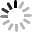February 3, 2023

## What are NTC Thermistors?

NTC stands for "Negative Temperature Coefficient". NTC thermistors are resistors with a negative temperature coefficient, which means that the resistance decreases with increasing temperature. They are primarily used as resistive temperature sensors and current-limiting devices. The temperature sensitivity coefficient is about five times greater than that of silicon temperature sensors (silistors) and about ten times greater than that of resistance temperature detectors (RTDs). NTC sensors are typically used in a range from −55 to +200 °C.

The non-linearity of the relationship between resistance and temperature exhibited by NTC resistors posed a great challenge when using analog circuits to accurately measure temperature. However, rapid development of digital circuits solved that problem through enabling computation of precise values by interpolating lookup tables or by solving equations which approximate a typical NTC curve.